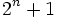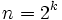Difference between revisions of "Fermat prime"

This article defines a property that can be evaluated for a prime number. In other words, every prime number either satisfies this property or does not satisfy this property.
View other properties of prime numbers | View other properties of natural numbers

Definition

A Fermat prime is a Fermat number that is also a prime number. In other words, it is a prime number of the form, whereis a nonnegative integer.

It turns out that ifis prime fora natural number, thenfor some nonnegative integer. Thus, a Fermat prime can also be defined as a prime of the formfor some natural number.

Testing

The ID of the sequence in the Online Encyclopedia of Integer Sequences is A019434